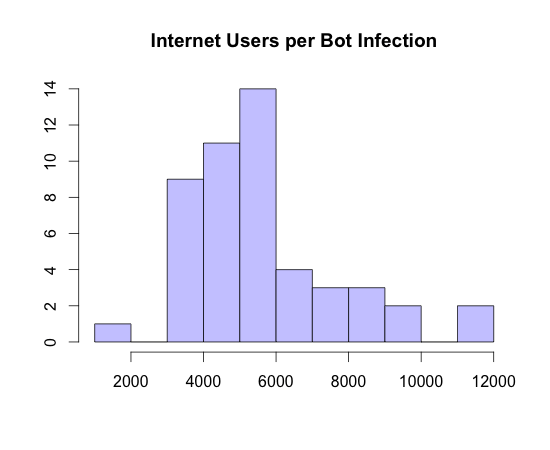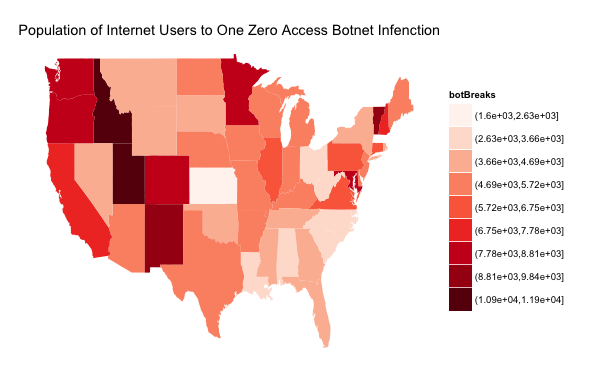Want to share your content on R-bloggers? click here if you have a blog, or here if you don't.

NOTE: A great deal of this post comes from @jayjacobs as he took a conversation we were having about thoughts on ways to look at the data and just ran like the Flash with it.

Did you know that – if you’re a US citizen – you have approximately a 1 in 5 chance of getting the flu this year? If you’re a male (no regional bias for this one), you have a 1 in 400 chance of developing Hodgkin’s Disease and a 1 in 5,000 chance of dying from testicular cancer.

Moving away from medical stats, if you’re a NJ resident, you have a 1 in 1,000 chance of winning $275 in the straight “Pick 3″ lottery and a 1 in 13,983,816 chance of jackpotting the “Pick 6″. What does this have to do with botnets? Well, we’ve determined that – if you’re a US resident – you have a 1 in 6,000 chance of getting the ZeroAccess flu (or winning the ZeroAccess lottery, whichever makes you feel better). Don’t believe me? Let’s look at the data. For starters, we’re working with this file which is a summary file by US state that includes actual state population, the number of internet users in that state and the number of bots in that state (data is from Internet World Statistics). As an example, Maine has: • 1,332,155 residents • 1,102,933 internet users • 219 bot infections (To aspiring security data scientists out there, I should point out that we’ve had to gather or crunch through on our own much of the data we’re using. While @fsecure gave us a great beginning, there’s no free data lunch) Where’d we get the 1 : 6000 figure? We can do some quick R math and view the histogram and summary data: #read in the summary data df <- read.csv("zerogeo.csv", header=T) # calculate how many people for 1 bot infection per state: df$per <- round(df$intUsers/df$bots)   # plot histogram of the spread hist(df$per, breaks=10, col="#CCCCFF", freq=T, main="Internet Users per Bot Infection")Along with the infection rate/risk, we can also do a quick linear regression to see if there’s a correlation between the number of internet users in a state and the infection rate of that state: # "lm" is an R function that, amongst other things, can be used for linear regression # so we use it to performa quick regression on how internet users describe bot infections users <- lm(df$bots~df$intUsers) # and, R makes it easy to plot that model plot(df$intUsers, df$bots, xlab="Internet Users", ylab="Bots", pch=19, cex=0.7, col="#3333AA") abline(users, col="#3333AA")Apart from some outliers (more on that in another post), there is – as Jay puts it – “very strong (statistical) relationship between the population of internet users and the infection rate in the states.” Some of you may be saying “Duh?!” right about now, but all we’ve had up until this point are dots or colors on a map. We’ve taken that superficial view (yes, it’s just really eye candy) and given it some depth and meaning. We’re pulling some demographic data from the US Census and will be doing another data summarization at the ZIP code level to see what other aspects (I’m really focused on analyzing median income by ZIP code to see if/how that describes bot presence). If you made it this far, I’d really like to know what you would have thought the ZeroAccess “flu” chances were before seeing that it’s 1 : 6,000 (since your guesstimate was probably based on the map views). Finally, Jay used the summary data to work up a choropleth in R: # setup our environment library(ggplot2) library(maps) library(colorspace) # read the data zero <- read.csv("zerogeo.csv", header=T) # extract state geometries from maps library states <- map_data("state") # this "cleans up the data" to make it easier to merge with the built in state data zero.clean <- data.frame(region=tolower(zero$state), perBot=round(zero$intUsers/zero$bots), intUsers=zero$intUsers) choro <- merge(states, zero.clean, sort = FALSE, by = "region") choro <- choro[order(choro$order),]   # "bin" the data to enable us to use a better set of colors choro$botBreaks <- cut(choro$perBot, 10)   # get the plot c1 = qplot(long, lat, data = choro, group = group, fill = botBreaks, geom = "polygon", main="Population of Internet Users to One Zero Access Botnet Infenction") + theme(axis.line=element_blank(),axis.text.x=element_blank(), axis.text.y=element_blank(),axis.ticks=element_blank(), axis.title.x=element_blank(), axis.title.y=element_blank(), panel.background=element_blank(),panel.border=element_blank(),panel.grid.major=element_blank(), panel.grid.minor=element_blank(),plot.background=element_blank())   # display it with modified color scheme (we hate the default ggplot2 blue) c1 + scale_fill_brewer(palette = "Reds")